• 鸢尾花卉数据集，是一类多重变量分析的数据集。通过花萼长度，花萼宽度，花瓣长度，花瓣宽度4个属性预测鸢尾花卉属于（Setosa，Versicolour，Virginica）三个种类中的哪一类。是机器学习基础学习的典型案例。
• 两行代码获取鸢尾花的数据。 我们要进行的是有监督的训练，同样在学习的朋友可以先查看一下 “iris = load_iris()” iris的数据格式，对我们有用的是 data,target 两个数据，各有150条，每50条为一类，d...
安装好sklearn这个库之后，就可以直接
from sklearn.datasets import load_iris


两行代码获取鸢尾花的数据。
我们要进行的是有监督的训练，同样在学习的朋友可以先查看一下 “iris = load_iris()” iris的数据格式，对我们有用的是 data,target 两个数据，各有150条，每50条为一类，data是它的数据，target是对应的类别。 了解数据格式后，直接进入实现部分。
线性回归：
一、
我们先从数据中的各类别取出一部分数据进行训练
indexs = random.sample(range(0,50),30) + random.sample(range(50,100),30) +random.sample(range(100,150),30)

二、
将选择的数据和其对应的类别 使用 LinearRegression 的 fit 函数进行计算出对应的系数
for index in indexs:
X.append( iris.data[index] )
y.append( iris.target[index] )
lr.fit(X,y)   # lr = LinearRegression(fit_intercept=False)

print(lr.coef_,'\n\n\n') #输出对应的系数


三、
带点进去，观察值的范围
coefficients = lr.coef_  #系数的集合
for i in range(60,70):
y = 0
for j in range(0,4):
y += (coefficients[j] * iris.data[i][j] )
print(y,'\n')

结果如图所示：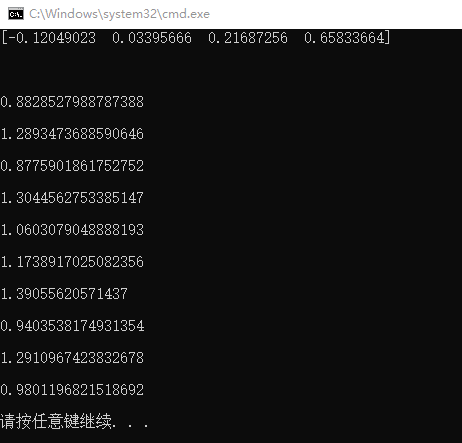都在1之间波动。
四、
自己定一个规则，然后进行预测 我是直接四舍五入
indexs = random.sample(range(0,150),150)
count = 0
for index in indexs:
y = 0
for j in range(0,4):
y += (coefficients[j] * iris.data[index][j] )
print( round(y) ,end=' ')
if round(y) == iris.target[index]:
print('预测正确')
count += 1
else :
print('预测错误')

accuracyRate = count/150*100

print('正确率为：',accuracyRate,'%')


展开全文逻辑回归
• 本次实验我们做二分类任务，鸢尾花数据集有三个分类，那我们需要选择两个类。 逻辑回归内部也使用梯度下降算法 数据集资源 本文基于鸢尾花 数据集实现 数据集：数据集网盘下载 提取码：p2v9 数据集简单处理 import ...
注意
逻辑回归是分类模型！！！ 本次实验我们做二分类任务，鸢尾花数据集有三个分类，那我们需要选择两个类。
逻辑回归内部也使用梯度下降算法
数据集资源
本文基于鸢尾花 数据集实现 数据集：数据集网盘下载 提取码：p2v9
数据集简单处理
import numpy as np
import pandas as pd

data = pd.read_csv(r"dataset/iris.arff.csv")
#data
# 删除重复记录
data.drop_duplicates(inplace=True)
#  Iris-setosa Iris-versicolor Iris-virginica
data["class"] = data["class"].map({"Iris-versicolor":0,"Iris-setosa":1,"Iris-virginica":2 })
# 只选取类别为0与1的鸢尾花数据，进行逻辑回归的二分类
data = data[data["class"] != 2]
# len(data) #98

逻辑回归代码实现
class logisticRegression:
"""使用python语言实现逻辑回归算法"""

def __init__(self,alpha,times):
"""初始化方法

Parameters：
alpha：float
学习率

times：int
迭代次数

"""
self.alpha = alpha
self.times = times

def sigmoid(self,z):
"""sigmoid函数的实现

Parameters：
z：float
自变量，值为z = w.T * x

Return:

p: float，值为[0,1]之间
返回样本属于类别1的概率值，用来作为结果的预测。
当x>=0.5(z>=0)，判定为类别1；否则，判定为类别0.

"""

return 1.0 / (1.0 + np.exp(-z))

def fit(self,X,y):
"""根据提供的训练数据，对模型进行训练。

Parameters：
X：类数组类型。形状为：[样本数量，特征数量]
待训练样本的特征属性矩阵。

y：类数组类型。形状为：[样本数量]
每个样本的目标值。（标签）

"""

X = np.asarray(X)
y = np.asarray(y)

#初始化权重向量，注意：w_的长度比特征数多1（为截距）。
self.w_ = np.zeros(1 + X.shape)
# 创建损失列表，用来保存每次迭代后的损失值
self.loss_ = []

for i in range(self.times):
z = np.dot(X,self.w_[1:]) + self.w_
# 计算概率值
p = self.sigmoid(z)
# 根据逻辑回归的代价函数，计算损失值
# 逻辑回归的目标函数
# J(w) = -sum(yi * log(s(zi))+(1-yi)*log(1-s(zi))) {i从1到n,n为样本数量}
cost = -np.sum(y * np.log(p) + (1 - y)*np.log(1-p))
self.loss_.append(cost)

# 调整权重值,根据公式调整为：权重(j列) = 权重(j列) + 学习率*sum((y - s(z))*x(j))
self.w_ += self.alpha * np.sum(y - p)
self.w_[1:] += self.alpha * np.dot(X.T,y-p)

def predict_proba(self,X):
"""根据参数传递的样本，对样本数据进行预测。

Parameters：
X：类数组类型。形状为：[样本数量，特征数量]
待测试样本特征（属性）

Return:
result：数组类型
预测的结果（概率值）

"""

X = np.asarray(X)
z = np.dot(X,self.w_[1:]) + self.w_
p = self.sigmoid(z)
# 将预测结果变成二维结构，便于后续的拼接
p = p.reshape(-1,1)
# 将2个数组进行拼接,方向是横向的拼接
return np.concatenate([1-p,p],axis=1)

def predict(self,X):
"""根据参数传递的样本，对样本数据进行预测。
Parameters：
X：类数组类型。形状为：[样本数量，特征数量]
待测试样本特征（属性）

Return:
result：数组类型
预测的结果（类别）

"""
return np.argmax(self.predict_proba(X),axis=1)

数据打散&构建训练集与测试集
t1 = data[data["class"] == 0]
t2 = data[data["class"] == 1]
t1 = t1.sample(len(t1),random_state=0)
t2 = t2.sample(len(t2),random_state=0)

train_X = pd.concat([t1.iloc[:40,:-1], t2.iloc[:40,:-1]],axis=0)
train_y = pd.concat([t1.iloc[:40,-1], t2.iloc[:40,-1]],axis=0)

test_X = pd.concat([t1.iloc[40:,:-1], t2.iloc[40:,:-1]],axis=0)
test_y = pd.concat([t1.iloc[40:,-1], t2.iloc[40:,-1]],axis=0)


训练&预测
注意：虽然应用了梯度下降算法，但与上一讲【python机器学习】线性回归–梯度下降实现（基于波士顿房价数据集） 不同的是，鸢尾花数据集每个特征列的数量级都一样，所以不用标准化。
lr = logisticRegression(alpha=0.01,times=20)
lr.fit(train_X,train_y)

预测的概率值
#预测的概率值
lr.predict_proba(test_X)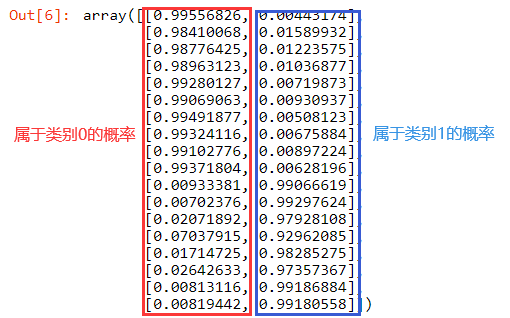预测的类别
#预测的类别
lr.predict(test_X)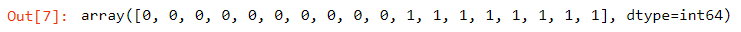计算准确度
#计算准确度
result = lr.predict(test_X)
print(np.sum(result == test_y)) #18个
print(np.sum(result == test_y)/len(test_y))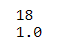可视化
import matplotlib as mpl
import matplotlib.pyplot as plt
mpl.rcParams["font.family"] = "SimHei"
mpl.rcParams["axes.unicode_minus"] = False

# 绘制预测值
plt.plot(result,"ro",ms=15,label="预测值")
# 绘制真实值
plt.plot(test_y.values,"go",label="真实值")
plt.title("逻辑回归")
plt.xlabel("样本序号")
plt.ylabel("类别")
plt.legend()
plt.show()
# 发现全部"中靶"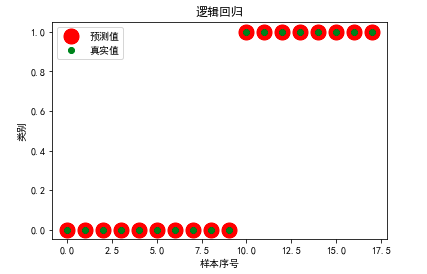发现全部"中靶"
绘制损失值
# 绘制损失值
plt.plot(range(1,lr.times+1),lr.loss_,"go-")
plt.xlabel("迭代次数")
plt.ylabel("损失值")
plt.show()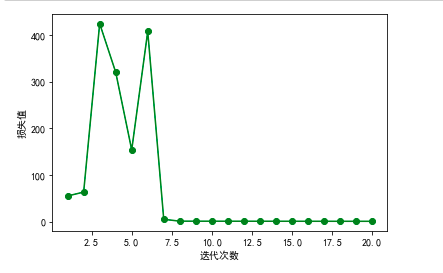展开全文python 机器学习
• 归一化： Python数据预处理—归一化，标准化，正则化_dengheng4891的博客-CSDN博客
归一化： Python数据预处理—归一化，标准化，正则化_dengheng4891的博客-CSDN博客混淆矩阵： 混淆矩阵及其可视化 - 知乎数据集导入： 机器学习“HelloWorld！”——鸢尾花的分类【完整版】_RickieLim的博客-CSDN博客展开全文机器学习 iris
• 基于鸢尾花数据集实现线性判别式多分类 本文在自己编写梯度下降的逻辑斯蒂判别式算法的基础上，对鸢尾花数据集实现多分类。 鸢尾花数据集公包含三类数据，每条数据四个特征，从中随机选取70%的数据作为训练集，30%的...
• 什么是线性回归 线性回归模型——将数据拟合成一条直线。 导入Iris鸢尾花数据集 Iris鸢尾花数据集共有150条记录，分别是50条山鸢尾 (Iris-setosa)、50条变色鸢尾(Iris-versicolor)、50条维吉尼亚鸢尾(Iris-virginica...


文章目录
一、具体实现步骤1. 导入Iris鸢尾花数据集2. 提取花瓣数据3. 拆分数据4. 训练模型
二、可视化结果展示1. 训练集2. 测试集
三、相关知识点讲解1. train_test_split()函数2. LinearRegression()函数3. 散点图与折线统计图的绘制

这篇文章中，我们要通过鸢尾花的花瓣长度预测花瓣宽度
环境：Python3.6.5编译器：jupyter notebook代码|数据：微信公众号（明天依旧可好）中回复：第1天
一、具体实现步骤
1. 导入Iris鸢尾花数据集
Iris鸢尾花数据集共有150条记录，分别是：
50条山鸢尾 (Iris-setosa)50条变色鸢尾(Iris-versicolor)50条维吉尼亚鸢尾(Iris-virginica)
url = "https://archive.ics.uci.edu/ml/machine-learning-databases/iris/iris.data"
names = ['花萼-length', '花萼-width', '花瓣-length', '花瓣-width', 'class']

2. 提取花瓣数据
下面我们提取数据集中花瓣宽度与花瓣长度数据，将花瓣数据分为训练数据与测试数据，训练数据用于训练线性回归模型，测试数据用于检测我们的模型的准确率。
最终我们要达到的效果是：输入花瓣宽度，通过模型预测花瓣宽度。
X = dataset["花瓣-length"]
Y = dataset["花瓣-width"]
X = X.reshape(len(X),1)
Y = Y.reshape(len(Y),1)

3. 拆分数据
将数据集拆分数据集成训练集、测试集
from sklearn.model_selection import train_test_split
X_train, X_test, Y_train, Y_test = train_test_split(X, Y, test_size=0.2, random_state=0)

4. 训练模型
这里我们需要将我们的训练数据喂给模型进行训练。
from sklearn.linear_model import LinearRegression
regressor = LinearRegression()
regressor = regressor.fit(X_train, Y_train)

二、可视化结果展示
1. 训练集
将训练集中每一朵花的花瓣数据与线性回归模型预测的结果放到同一张统计图中。
import matplotlib.pyplot as plt

plt.scatter(X_train, Y_train, color='red')
plt.plot(X_train, regressor.predict(X_train), color='green')
plt.xlabel("Iris-length")
plt.ylabel("Iris-width")
plt.title("This is train dataset-kzb")
plt.show()

红色的点是训练数据集中的花瓣数据，我们不难看出花瓣长度与宽度是一个线性关系，绿色的线是我们模型拟合的结果。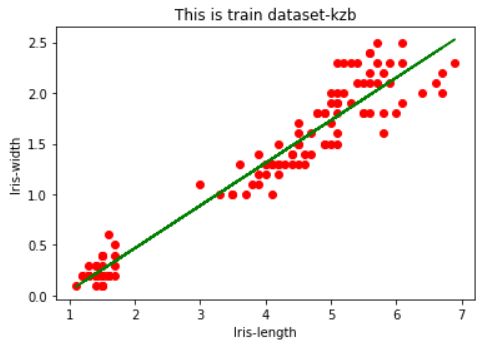2. 测试集
将测试集中每一朵花的花瓣数据与线性回归模型预测的结果放到同一张统计图中。
plt.scatter(X_test, Y_test, color='blue')
plt.plot(X_train, regressor.predict(X_train), color='green')
plt.xlabel("Iris-length")
plt.ylabel("Iris-width")
plt.title("This is test dataset-kzb")
plt.show()

绿色的点是测试数据集中的花瓣数据，我们可以看出这部分数据也是符合线性关系的，随着集的增大，线性关系会更加明显。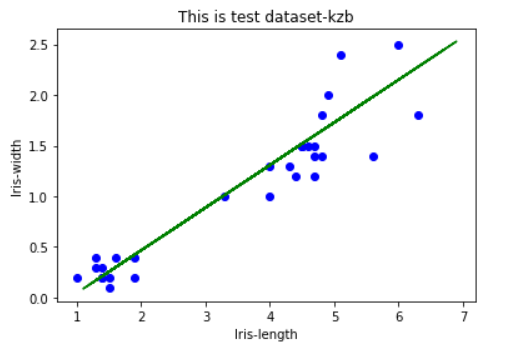三、相关知识点讲解
1. train_test_split()函数
train_test_split()：将数据集划分为测试集与训练集。
X：所要划分的整体数据的特征集；Y：所要划分的整体数据的结果；test_size：测试集数据量在整体数据量中的占比（可以理解为X_test与X的比值）；random_state：①若不填或者填0，每次生成的数据都是随机，可能不一样。②若为整数，每次生成的数据都相同；
from sklearn.model_selection import train_test_split
X_train, X_test, Y_train, Y_test = train_test_split(X, Y, test_size=0.2, random_state=0)

2. LinearRegression()函数
sklearn.linear_model包实现了广义线性模型，包括线性回归、Ridge回归、Bayesian回归等。LinearRegression是其中较为简单的线性回归模型。
解释一下什么是回归：回归最简单的定义是，给出一个点集D，用一个函数去拟合这个点集，并且使得点集与拟合函数间的误差最小，如果这个函数曲线是一条直线，那就被称为线性回归，如果曲线是一条二次曲线，就被称为二次回归。
3. 散点图与折线统计图的绘制
plt.scatter()：绘画出数据的散点图 plt.plot()：绘画出依据模型（LinearRegression的线性回归模型）生成的直线
更详细的介绍可以参考：【Matplotlib可视化系列教程】

【机器学习100天目录】 【机器学习第2天：线性回归（理论篇）】 【机器学习第3天：预测汽车的燃油效率】 【机器学习第4天：预测1立方米混凝土抗压强度】 【机器学习第5天：逻辑回归】
如有错误欢迎指教，有问题的也可以加入QQ群（1149530473）向我提问，关注微信公众号（明天依旧可好）和我同步。
展开全文机器学习
• # encoding: utf-8 from sklearn.linear_model import LogisticRegression import numpy as np from sklearn import model_selection import matplotlib.pyplot as plt import matplotlib as mpl ...
• 鸢尾花数据集可视化、线性回归、决策树花样分析 1.鸢尾花数据集可视化分析 2.线性回归分析鸢尾花花瓣长度和宽度的关系 3.决策树分析鸢尾花数据集 4.Kmeans聚类分析鸢尾花数据集 一. 鸢尾花数据集介绍 本章采用Pyt....
• 文章目录 ‘鸢尾花数据集’ 一、实验说明 二、LogisticRegression回归模型在Sklearn中的使用 三、鸢尾花数据集线性多分类 四、总结 ‘鸢尾花数据集’ Iris flower数据集是1936年由Sir Ronald Fisher引入的经典多维...
• 1、介绍 前言   为了更好的理解本章内容，请参考下面的网址，自主学习一些矩阵...  逻辑回归（Logistic Regression）虽然是名词是回归，但是实际上它是一种分类模型。假设输入值函数（单值x1x_1x1​）如下： z=WTpython 机器学习
• 线性回归推导： 上图求导部分有误,少些一个转置符号，更正为： 逻辑回归推导： （公式中“ln”和“log”表示一个意思，都是以“e”为低的自然对数）： 公式中：X是m*n矩阵，m个样本，n维特征。 1、内容...sklearn iris Ridge regression
• 2、线性分类器的实现原理3、设计线性分类器的主要步骤4、Fisher线性判别二、例题1–鸢尾花数据集的分类可视化及预测1、准备鸢尾花数据集2、打开jupyter进行python环境创建3、编写鸢尾花数据集的分类可视化代码4、...
• 鸢尾花分类问题时一个很经典的问题，我就想从这个问题入手吧。网上有一些相关的代码，但是我看到的几 个都有些肉眼可见的缺陷，所以，我索性把网上的参考抛开，按照自己的思路实现一个。 会有不少缺陷，求 大神轻喷 ...
• 文章目录前言一、逻辑回归模型1.1模型简介1.2 算法的分类思想1.3sigmoid函数1.4逻辑回归损失函数二.逻辑回归实现二分类2.1模型训练与预测2.2计算概率2.3绘制决策边界三、逻辑回归实现多分类 前言 本篇主要学习逻辑...python 机器学习 可视化
• 鸢尾花数据集为机器学习常用的数据集，今天，我们基于该数据集进行算法学习 鸢尾花数据集 鸢尾花数据集有3个类别，每个类别有50个样本，其中一个类别与另外两个线性可分，另外两个线性不可分 特征： sepal_length...
• 线性回归算法实验 一、什么是线性回归。 线性回归就是用一条直线来解释自变量与因变量之间的关系。机器学习 python
• 0x1：线性回归和线性分类中的“线性”指的是什么 线性(linear)，指量与量之间按比例、成直线的关系，在数学上可以理解为一阶导数为常数的函数；非线性(non-linear)则指不按比例、不成直线的关系，一阶导数不为常数 ...
• 该数据集的四个特征属性的取值都是数值型的，他们具有相同的量纲，不需要你做任何标准化的处理，第五列为通过前面四列所确定的鸢尾花所属的类别名称。 type ( iris ) # sklearn.utils.Bunch 数据集长这样： ...机器学习
• 应用逻辑回归方法对鸢尾花进行分类 1. 实验目标 逻辑回归 了解Iris数据集 2. 本次实验主要使用的 ????????????ℎ????????Python 库 名称 版本 简介 ????????????????????numpy 1.16.21.16.2 线性代数 ??...
• ## 基于鸢尾花lr分类 import pandas as pd import numpy as np import matplotlib.pyplot as plt import seaborn as sns from sklearn.datasets import load_iris iris = load_iris() iris_target = iris.target ...
• 自然界存在着大量线性关系的数据，线性关系的表达在数学里 用python表达用python表达用python表达，称为内敛模式.行内公式，python表达python表达python表达块模式，独占一行居中 这就是线性关系的表达 这种表达...
• 本文主要讲述鸢尾花数据集的各种分析，包括可视化分析、线性回归分析、决策树分析等，通常一个数据集是可以用于多种分析的，希望这篇文章对大家有所帮助，同时提供些思考。内容包括： 1.鸢尾花数据集可视化分析 2....数据挖掘 数据分析 决策树
• 用matlab实现用Bp神经网络对iris数据集进行分类（以及影响分类性能的参数条件）这篇文章的数据集
• 线性回归算法 **一般都是准备数据，训练，可视化。 导入x数据和y数据。 ** 产生一个空的数组，把x_data里的数据语言，有多少个数据（取0行），列数增加1，保留数据值再加上dtype，np.float浮点数。 Y值，本来是...
• Python实现线性回归、逻辑回归、KNN、SVM、朴素贝叶斯、决策树、K-Means7种机器学习算法的经典案例——亲测可用
• Iris逻辑回归分类 from sklearn.model_selection import train_test_split import matplotlib.pyplot as plt import numpy as np import pandas as pd # Data load iris = pd.read_csv('./Iris/iris.data') # Data...iris 随机梯度下降 python
• 一元线性回归练习二、四重奏数据集分析三、小结 一、线性回练习 1. 一元线性回归练习 高尔顿数据集进行线性回归分析 父母身高平均值和其中一个子女身高进行回归分析 数据整理，一般获得的数据都会比较多，必须先...机器学习
• import numpy as np import pandas as pd import matplotlib as mpl import matplotlib.pyplot as plt from sklearn.datasets import data pd.set_option("display.max_rows",None) data = pd.read_csv(r"D:\...python 机器学习 可视化...